Monday, September 28, 2020
Home > NTSE > NTSE Stage 1 Mock Test Papers for SAT Maths

# NTSE Stage 1 Mock Test Papers for SAT Maths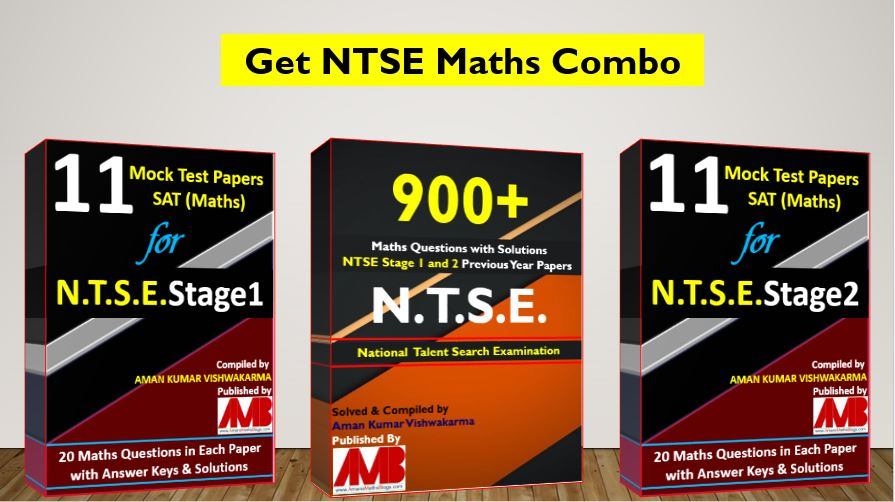# NTSE Stage 1 Mock Test Papers for SAT Maths

Hi Students, welcome to Amans Maths Blogs (AMB). On this blog post, you will get the NTSE Stage 1 Mock Test Papers for SAT Maths. These mock test papers are sample papers for NTSE Stage 1. As you know that, this maths blogs AMB is a special blogs for maths subjects. Thus, these mock test papers consisting only Maths questions, which help to practice for NTSE Stage 1 SAT (Maths) Papers.

According to official website NCERT, the syllabus for SAT (Maths) paper of NTSE Stage 1 and 2, the questions asked are as: Arithmetic Progression, Circle, Congruent Triangles, Coordinate Geometry, Exponents, Height & Distance, Linear Equations, Logarithms, Mensuration, Number System, Polynomial, Probability, Quadratic Equations, Quadrilateral, Real Numbers, Shaded Regions, Similar Triangles, Statistics, Straight Lines, Tangent To Circle, Trigonometry.

Thus, on this post, I have complied 11 Mock Test Papers for NTSE Stage 1 consisting only Maths questions. Each Mock Test Papers for NTSE contains 20 maths questions along with answer keys and its solutions. All the questions are based on the above topics of Maths that is the syllabus of NTSE.

Get File: Free PDF of 129 Maths Short Tricks (Click On Image)## NTSE Stage 1 Mock Test Paper 1 : SAT (Maths)

NTSE Stage 1 Mock Test Papers for SAT Maths: Ques No 1:

If p(x) = (x+1)4 + 3(x+2)(x+6) + 8, then the sum of its possible real roots is

Options:

A. 0

B. -8

C. -6

D. 10

NTSE Stage 1 Mock Test Papers for SAT Maths: Ques No 2:

In the figure below, angle AOB = 74 degree. The bisectors OM and ON of ∠AOB and ∠BOC respectively is drawn. If the angles between the bisectors of ∠AON and ∠COM is 40°, then the value ∠BOC is (AOC is not a straight line).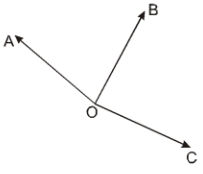Options:

A. 80 degree

B. 81 degree

C. 86 degree

D. 91 degree

NTSE Stage 1 Mock Test Papers for SAT Maths: Ques No 3:

In a triangle ABC, A = 80° and B = 70°, then find the value of sin2A + sin2B – sin2C.

Options:

A. 2cos10 – Sqrt(3)

B. cos10 – Sqrt(3)/2

C. cos10 + Sqrt(3)

D. cos10 + Sqrt(3)/2

NTSE Stage 1 Mock Test Papers for SAT Maths: Ques No 4:

If the coordinates of the mid points of a line segment AB is (–2, 1). If the coordinate of A is (8, 12), then the coordinate of B is

Options:

A. (11, 11)

B. (11, 12)

C. (-12, -10)

D. (-13, -12)

NTSE Stage 1 Mock Test Papers for SAT Maths: Ques No 5:

If the equations 2x + 3y = 7 and 2mx + (m + n)y = 28 have infinite number of solutions, then the value of n is

Options:

A. 4

B. 8

C. 2

D. 5

NTSE Stage 1 Mock Test Papers for SAT Maths: Ques No 6:

If both the roots of the equation x2 – 12x + k = 0 are prime numbers. Then the sum of the squares of the roots are

Options:

A. 74

B. 64

C. 94

D. 36

NTSE Stage 1 Mock Test Papers for SAT Maths: Ques No 7:

There are five natural numbers in ascending order. The mean of the five natural numbers is 75 and the mean of the last four numbers is 85, then the first number is

Options:

A. 32

B. 35

C. 38

D. 40

NTSE Stage 1 Mock Test Papers for SAT Maths: Ques No 8:

A sphere and cylinder has same radius and volume. Then, find the ratio of the radius of the cylinder to that of its height

Options:

A. 4 : 3

B. 3 : 2

C. 2 : 3

D. 3 : 4

NTSE Stage 1 Mock Test Papers for SAT Maths: Ques No 9:

Find the area of an equilateral triangle inscribed in a circle of radius (12)^(1/4) cm.

Options:

A. 4 cm2

B. 5.5 cm2

C. 5 cm2

D. 5.5 cm2

NTSE Stage 1 Mock Test Papers for SAT Math: Ques No 10:

If you have 3 tickets of a lottery for which 10 tickets were sold and 5 prizes are to be given, then the probability that you will win at least one prize is

Options:

A. 1/12

B. 11/12

C. 9/12

D. 7/12

NTSE Stage 1 Mock Test Papers for SAT Maths: Ques No 11:

In a trapezium ABCD, the value of x is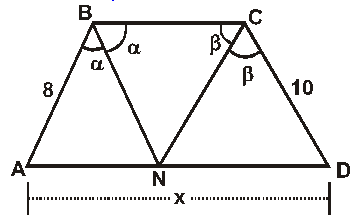Options:

A. 19

B. 17

C. 18

D. 12

NTSE Stage 1 Mock Test Papers for SAT Math: Ques No 12:

If the sum of first n terms of an AP is 3n2 – n, then the 31st term of the AP is

Options:

A. 381

B. 328

C. 182

D. 180

NTSE Stage 1 Mock Test Papers for SAT Math: Ques No 13:

Find the least number which when divided by 6, 7 and 9 leaves the remainders 1, 2 and 4 respectively.

Options:

A. 169

B. 144

C. 121

D. 165

NTSE Stage 1 Mock Test Papers for SAT Math: Ques No 14:

In the figure below, O is the centre of the circle. AB = 9, DE = 7 and BC = 8, the value of OE is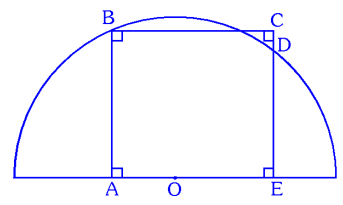Options:

A. 2

B. 4

C. 6

D. 8

NTSE Stage 1 Mock Test Papers for SAT Math: Ques No 15:

In the figure below, ABCD is a square and APQ is the equilateral. The ratio of the regions S1 to S2 is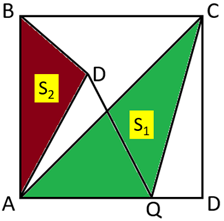Options:

A. 1 : 1

B. 2 : 1

C. 4 : sqrt(3)

D. sqrt(3) : 1

NTSE Stage 1 Mock Test Papers for SAT Math: Ques No 16:

In the figure below, AB = BE and BC = BD, then the value of x isOptions:

A. 30 degree

B. 45 degree

C. 15 degree

D. 20 degree

NTSE Stage 1 Mock Test Papers for SAT Math: Ques No 17:

ABCD is a parallelogram in which angle A = 45° and O is the intersection point of its diagonals. If the distances of O from the sides AD and CD are 4 cm and 6 cm, then the area of the parallelogram ABCD is

Options:

A. 96Root(2) cm2

B. 76Root(2) cm2

C. 68Root(2) cm2

D. 72Root(2) cm2

NTSE Stage 1 Mock Test Papers for SAT Math: Ques No 18:

The angle of elevation of the top of a tower at a point on the ground is 30°. If on walking 20 meters towards the tower, the angle of elevation become 60°, then the height of the tower is

Options:

A. 10 m

B. 10/sqrt(3) m

C. 10sqrt(3) m

D. None of these

NTSE Stage 1 Mock Test Papers for SAT Math: Ques No 19:

The value of log4/3(1.333…)  is

Options:

A. 1

B. -1

C. 0

D. 2

NTSE Stage 1 Mock Test Papers for SAT Math: Ques No 20:

The simplification of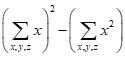Options:The Answer Keys & Solutions of this NTSE Stage 1 Mock Test Paper 1 for SAT (Maths) are in PDF file. To get this, click below RED button.NTSE Stage 1 Mock Test Paper 1 SAT (Maths): Paper 1 (FREE)

## NTSE Stage 1 Mock Test Paper 2 : SAT (Maths)

The Answer Keys & Solutions of this NTSE Stage 1 Mock Test Paper 2 for SAT (Maths) are in PDF file. To get this, click below RED button.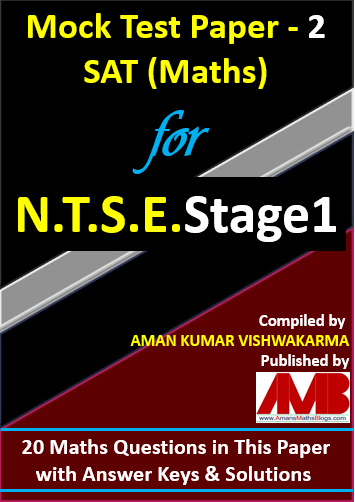NTSE Stage 1 Mock Test Paper 2 SAT (Maths): Paper 2 (Rs. 25)

## NTSE Stage 1 Mock Test Paper 3 : SAT (Maths)

The Answer Keys & Solutions of this NTSE Stage 1 Mock Test Paper 3 for SAT (Maths) are in PDF file. To get this, click below RED button.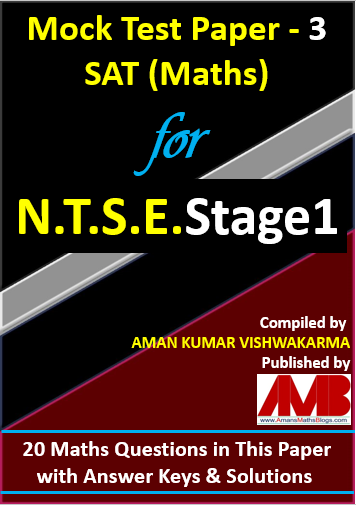NTSE Stage 1 Mock Test Paper 3 SAT (Maths): Paper 2 (Rs. 25)

## NTSE Stage 1 Mock Test Paper 4 : SAT (Maths)

The Answer Keys & Solutions of this NTSE Stage 1 Mock Test Paper 4 for SAT (Maths) are in PDF file. To get this, click below RED button.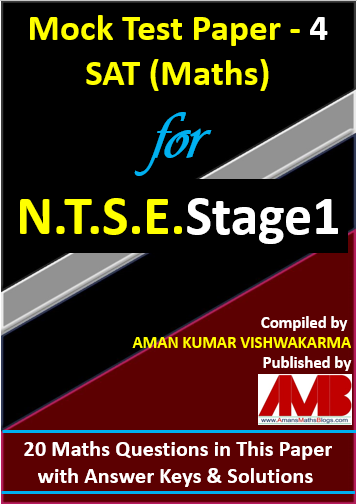NTSE Stage 1 Mock Test Paper 4 SAT (Maths): Paper 2 (Rs. 25)

## NTSE Stage 1 Mock Test Paper 5 : SAT (Maths)

The Answer Keys & Solutions of this NTSE Stage 1 Mock Test Paper 5 for SAT (Maths) are in PDF file. To get this, click below RED button.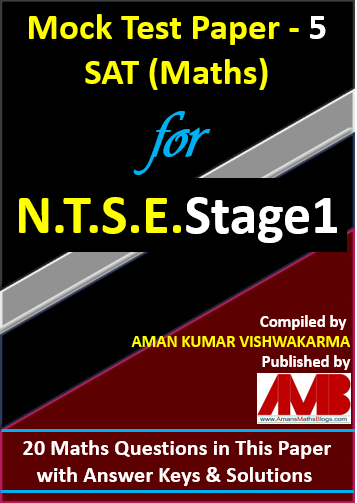NTSE Stage 1 Mock Test Paper 5 SAT (Maths): Paper 2 (Rs. 25)

## NTSE Stage 1 Mock Test Paper 6 : SAT (Maths)

The Answer Keys & Solutions of this NTSE Stage 1 Mock Test Paper 6 for SAT (Maths) are in PDF file. To get this, click below RED button.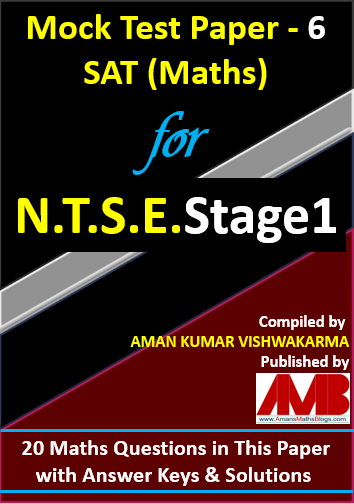NTSE Stage 1 Mock Test Paper 6 SAT (Maths): Paper 2 (Rs. 25)

## NTSE Stage 1 Mock Test Paper 7 : SAT (Maths)

The Answer Keys & Solutions of this NTSE Stage 1 Mock Test Paper 7 for SAT (Maths) are in PDF file. To get this, click below RED button.NTSE Stage 1 Mock Test Paper 7 SAT (Maths): Paper 2 (Rs. 25)

## NTSE Stage 1 Mock Test Paper 8 : SAT (Maths)

The Answer Keys & Solutions of this NTSE Stage 1 Mock Test Paper 8 for SAT (Maths) are in PDF file. To get this, click below RED button.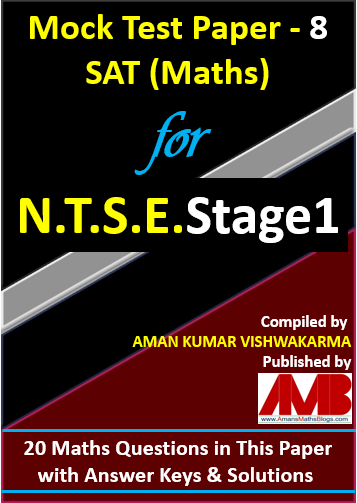NTSE Stage 1 Mock Test Paper 8 SAT (Maths): Paper 2 (Rs. 25)

## NTSE Stage 1 Mock Test Paper 9 : SAT (Maths)

The Answer Keys & Solutions of this NTSE Stage 1 Mock Test Paper 9 for SAT (Maths) are in PDF file. To get this, click below RED button.NTSE Stage 1 Mock Test Paper 9 SAT (Maths): Paper 2 (Rs. 25)

## NTSE Stage 1 Mock Test Paper 10 : SAT (Maths)

The Answer Keys & Solutions of this NTSE Stage 1 Mock Test Paper 10 for SAT (Maths) are in PDF file. To get this, click below RED button.NTSE Stage 1 Mock Test Paper 10 SAT (Maths): Paper 2 (Rs. 25)

## NTSE Stage 1 Mock Test Paper 11 : SAT (Maths)

The Answer Keys & Solutions of this NTSE Stage 1 Mock Test Paper 11 for SAT (Maths) are in PDF file. To get this, click below RED button.NTSE Stage 1 Mock Test Paper 11 SAT (Maths): Paper 2 (Rs. 25)

## NTSE Mock Test Paper Combined (1 to 11): SAT (Maths)

The Question Paper and its Answer Keys & Solutions of this NTSE Mock Test Paper 1 SAT (Maths) are in PDF file. To get this, click below RED button.Total cost of all 10 Mock Test Papers for NTSE Stage 1 is 10xRs25 = Rs 250. But if you get all 11 Mock Test Papers for NTSE Stage 1, you will get 52% off.

11 Mock Test Paper for NTSE Stage 1 SAT (Maths) (52% Off)

# NTSE Stage 2 Mock Test Papers for SAT Maths

I have complied 11 NTSE Stage 2 Mock Test Papers for SAT Maths consisting only Maths questions. Each Mock Test Papers for NTSE contains 20 maths questions along with answer keys and its solutions. All the questions are based on topics of Maths that is the syllabus of NTSE. To get the NTSE Stage 2 Mock Test Papers for SAT Maths, Click Here.

# NTSE Maths Combo EBook

Get NTSE Maths Previous Year Question Bank with 900+ Questions and its Solutions and 11 Mock Test Papers for NTSE Stage 1 and 2 EBook. To get this combo ebook, click below RED button.NTSE Maths Questions Bank & 11 Mock Test Paper for NTSE Stage 1 & 2 SAT (Maths)

error: Content is protected !!